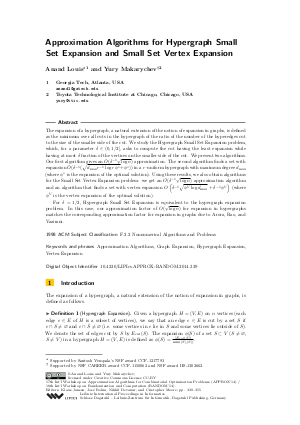Document# Approximation Algorithms for Hypergraph Small Set Expansion and Small Set Vertex Expansion

### Authors Anand Louis, Yury Makarychev## File

LIPIcs.APPROX-RANDOM.2014.339.pdf
• Filesize: 0.53 MB
• 17 pages

## Cite As

Anand Louis and Yury Makarychev. Approximation Algorithms for Hypergraph Small Set Expansion and Small Set Vertex Expansion. In Approximation, Randomization, and Combinatorial Optimization. Algorithms and Techniques (APPROX/RANDOM 2014). Leibniz International Proceedings in Informatics (LIPIcs), Volume 28, pp. 339-355, Schloss Dagstuhl - Leibniz-Zentrum für Informatik (2014)
https://doi.org/10.4230/LIPIcs.APPROX-RANDOM.2014.339

## Abstract

The expansion of a hypergraph, a natural extension of the notion of expansion in graphs, is defined as the minimum over all cuts in the hypergraph of the ratio of the number of the hyperedges cut to the size of the smaller side of the cut. We study the Hypergraph Small Set Expansion problem, which, for a parameter 's' such that 0 < s < 1/2, asks to compute the cut having the least expansion while having at most 's' fraction of the vertices on the smaller side of the cut. We present two algorithms. Our first algorithm gives a multiplicative polylogarithmic approximation. Our second algorithm gives a bound that is a function of the expansion of the hypergraph but is independent of the size of the hypergraph. Using these results, we also obtain similar guarantees for the Small Set Vertex Expansion problem.
##### Keywords
• Approximation Algorithms
• Graph Expansion
• Hypergraph Expansion
• Vertex Expansion

## Metrics

• Access Statistics
• Total Accesses (updated on a weekly basis)
0